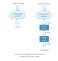# Understanding and Implementing Architectures of ResNet and ResNeXt for state-of-the-art Image Classification: From Microsoft to Facebook [Part 1]

## In this two part blog post we will explore Residual networks. More specifically we will discuss three papers released by Microsoft Research and Facebook AI research, state of the art Image classification networks- ResNet and ResNeXt Architectures and try to implement them on Pytorch.

This is Part 1 of two-part series explaining blog post exploring residual networks.

• Understanding and implementing ResNeXt Architecture[Part-2]

## Was ResNet Successful?

• Won 1st place in the ILSVRC 2015 classification competition with top-5 error rate of 3.57% (An ensemble model)
• Won the 1st place in ILSVRC and COCO 2015 competition in ImageNet Detection, ImageNet localization, Coco detection and Coco segmentation.
• Replacing VGG-16 layers in Faster R-CNN with ResNet-101. They observed a relative improvements of 28%
• Efficiently trained networks with 100 layers and 1000 layers also.

# What problem does ResNet solve?

## Problem:

When deeper networks starts converging, a degradation problem has been exposed: with the network depth increasing, accuracy gets saturated and then degrades rapidly.

Let us take a shallow network and its deeper counterpart by adding more layers to it.Shallow network and its deeper variant both giving the same output

## How to solve?

Instead of learning a direct mapping of x ->y with a function H(x) (A few stacked non-linear layers). Let us define the residual function using F(x) = H(x) — x, which can be reframed into H(x) = F(x)+x, where F(x) and x represents the stacked non-linear layers and the identity function(input=output) respectively.

The author’s hypothesis is that it is easy to optimize the residual mapping function F(x) than to optimize the original, unreferenced mapping H(x).

## Intuition behind Residual blocks:

If the identity mapping is optimal, We can easily push the residuals to zero (F(x) = 0) than to fit an identity mapping (x, input=output) by a stack of non-linear layers. In simple language it is very easy to come up with a solution like F(x) =0 rather than F(x)=x using stack of non-linear cnn layers as function (Think about it). So, this function F(x) is what the authors called Residual function.

## Test cases:

Take a plain network (VGG kind 18 layer network) (Network-1) and a deeper variant of it (34-layer, Network-2) and add Residual layers to the Network-2 (34 layer with residual connections, Network-3).

1. Down sampling with CNN layers with stride 2.
2. Global average pooling layer and a 1000-way fully-connected layer with Softmax in the end.

## Deeper Studies

The following networks are studied

## Observations:

1. ResNet Network Converges faster compared to plain counter part of it.
2. Identity vs Projection shorcuts. Very small incremental gains using projection shortcuts (Equation-2) in all the layers. So all ResNet blocks use only Identity shortcuts with Projections shortcuts used only when the dimensions changes.
3. ResNet-34 achieved a top-5 validation error of 5.71% better than BN-inception and VGG. ResNet-152 achieves a top-5 validation error of 4.49%. An ensemble of 6 models with different depths achieves a top-5 validation error of 3.57%. Winning the 1st place in ILSVRC-2015